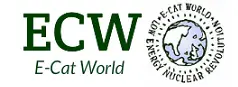# Rossi’s Spectrometry Based Power Calculation at the 10/24/2017 Demonstration (Michael Lammert)

The following post has been submitted by Michael Lammert

Rossi’s Spectrometry Based Power Calculation at the 10/24/2017 Demonstration
by Michael Lammert (aka Dr. Mike)

1/9/2018
Although Andrea Rossi was unable to complete a measurement of the output power of his 3 QX devices using spectrometry in the 11/24/2017 demonstration, he did make a calculation of the output power based on spectrometry data previously observed, which is described in the video of the demonstration from about 2:25:00 to 2:28:00. At about 2:27:45 Rossi asserts that “believe me, the final result will be about 70-71W”. How did Rossi arrive at 70-71W? The 70-71W does not agree with the 61W he calculated when he mistakenly used degrees C, rather than degrees K, in the Stefan-Boltzmann equation, or the ~40W when he did the calculation correctly.

I believe there was an error in his area calculation. At the 2:27:25 mark in the demonstration Rossi calculates the area (in cm^2) of the reactor as “2 times 0.08 times pi times 0.6”, whereas the actual area should have been “pi times 0.08 times 0.6” (πDL). (Note: In this calculation Rossi did specify the internal reactor diameter as 0.08cm and the length as 0.6cm, not mm.)

This means that Rossi’s calculation was off by a factor of 2, and therefore the result of his math in the presentation should have been “80-81W”, which would have been a factor of 2 greater than correct calculation of 40W. (Note: It has already been pointed out in comments on e-catworld that Rossi was neglecting that the spectrometer would have been measuring the output of 3 devices so the devices area should have been multiplied by a factor of 3.)

If the spectrometer measurement had been successful in the demonstration, would the measured spectrum have really shown an energy peak at a wavelength of 1.1μm? The answer is “no” assuming Rossi was really running 3 QX devices in parallel at 30% output power as he claimed. If it assumed that the full power temperature of reactor is 2636ºK (as claimed by Gullstrom in an earlier paper and Rossi in his JONP blog calculation), then the operating temperature of a reactor operating at 30% output power should be equal to 2636ºK divided by the 4th root of 1/0.3, or 1951ºK. The wavelength at the energy maximum on the spectrometer’s output should have been 2900μm-ºK / 1951ºK = 1.49μm (Wien’s Law). The total output power for the 3 QX devices would have been calculated from the Stefan-Boltzmann equation (assuming ɛ=1.0) as:
Pout = 3 x π x .08cm x 0.6cm x 5.67E-12W/cm^2/ºK^4 x (1951ºK)^4 = 37.2W

Does Rossi’s claim that the expected energy maximum for the spectrometer output should have been at a wavelength of 1.1μm mean that he hadn’t measured the devices with the spectrometer when operating at 30% power prior to the demonstration? The only other explanation for the claim that energy spectrum would show a peak at 1.1μm would be that the demonstration really consisted of a single device operating at full power. It’s too bad the spectrometer measurement in the demonstration didn’t work as it would have given some valuable information on how the demonstration was really run.

(Notes: 1) For those that might argue that a QX plasma temperature might really be 2636ºK when operating at 30% power, the full output power temperature would have to be 2636ºK times the 4th root of 1/0.3 or 3562ºK- way too hot! 2) An argument could be made that Rossi turned up the controller to full power for the spectrometry measurement. However, Rossi was very concerned with the QX devices over heating during this test, therefore it is unlikely that he would have made the over heating problem worse by turning up the power for this portion of the demonstration.)